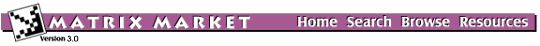BCSSTK30: BCS Structural Engineering Matrices (large eigenvalue problems)Statics module of an off-shore generator platform

from set BCSSTRUC5, from the Harwell-Boeing Collection

Stiffness matrix

Help: My browser can't read the compressed data files. What now?

Visualizations

Click on image to get an enlarged version. Click on label to get an explanation.

Matrix Statistics

* * * * * * * * * * * * * * * * * * * * * * * * * * * * * * * * * * * * * *
*                           Matrix Statistics                             *
*              Generated by Yousef Saad's SPARSKIT Library                *
* * * * * * * * * * * * * * * * * * * * * * * * * * * * * * * * * * * * * *
* * * * * * * * * * * * * * * * * * * * * * * * * * * * * * * * * * * * * *
* TIFFNESS MATRIX FOR OFF-SHORE GENERATOR PLATFORM (MSC NASTRAN) - PATTER *
*                    Key = BCSSTK30 , Type = PSA                          *
* No values provided - Information on pattern only                        *
* * * * * * * * * * * * * * * * * * * * * * * * * * * * * * * * * * * * * *
*  Dimension N                                      =      28924  *
*  Number of nonzero elements                       =    1036208  *
*  Average number of nonzero elements/Column        =    35.8252  *
*  Standard deviation for above average             =    15.2693  *
*  Nonzero elements in strict lower part            =    1007284  *
*  Nonzero elements in strict upper part            =    1007284  *
*  Nonzero elements in main diagonal                =      28924  *
*  Weight of longest column                         =        218  *
*  Weight of shortest column                        =          3  *
*  Matching elements in symmetry                    =          0  *
*  Relative Symmetry Match (symmetry=1)             = NaN         *
*  Average distance of a(i,j)  from diag.           =  0.454E+03  *
*  Standard deviation for above average             =  0.126E+04  *
*-----------------------------------------------------------------*
*  Lower bandwidth  (max: i-j, a(i,j) .ne. 0)       =          0  *
*  Upper bandwidth  (max: j-i, a(i,j) .ne. 0)       =      16947  *
*  Maximum Bandwidth                                =      16948  *
*  Average Bandwidth                                =  0.887E+03  *
*  Number of nonzeros in skyline storage            =   15715892  *
*  90% of matrix is in the band of width            =       1429  *
*  80% of matrix is in the band of width            =        803  *
*  The total number of nonvoid diagonals is         =       6619  *
*  The 10 most important diagonals are (offsets)    :             *
*     0     1     2     3     4     5     6     7     8     9     *
*  The accumulated percentages they represent are   :             *
*   2.8   5.3   7.6   9.9  12.1  14.3  16.3  18.2  19.9  21.5     *
*-----------------------------------------------------------------*
*  The matrix does not have a block structure                     *
*-----------------------------------------------------------------*

Set Information

Set BCSSTRUC5
Source: Boeing Computer Services
Discipline:Structural engineering
Accession:Spring 1986

The Matrix Market is a service of the Mathematical and Computational Sciences Division / Information Technology Laboratory / National Institute of Standards and Technology.

[ Home ] [ Search ] [ Browse ] [ Resources ]